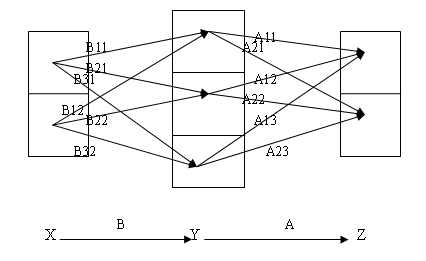# 图示矩阵分块乘法# 理解矩阵与矩阵乘积（二）

$$\varphi(u_1+u_2)=\varphi(u_1)+\varphi(u_2)$$
$$\varphi(ku)=k\varphi(u)$$

# 理解矩阵与矩阵乘积（一）

$$z=Ay, y=Bx$$

（待续）

# 从不同的角度看矩阵的行秩与列秩——兼论如何学好线性代数

A和A的共轭转置的列向量的秩分别对应于 T 和 T* 的值域的维度，能不能就此证明它们相等？从而至少可以证明实数矩阵行秩等于列秩。这就是下面的：

$$T^*v_1,T^*v_2,\dots,T^*v_s$$，考虑 $$TT^*v_1,TT^*v_2,\dots,TT^*v_s$$，这个向量组的秩≤s<r，因此可以在 $$T$$ 的值域中(维数为r)找到 $$v_{s+1}=Tu_{s+1}\not=0$$ 使得 $$\langle v_{s+1},TT^*v_i\rangle=\langle T^*v_{s+1},T^*v_i\rangle=0, i=1,2,\dots,s$$。又因为 $$\langle u_{s+1},T^*Tu_{s+1}\rangle=\langle Tu_{s+1},Tu_{s+1}\rangle\not=0$$ 故 $$T^*Tu_{s+1}\not=0$$ 即 $$T^*v_{s+1}\not=0$$。这样我们在 $$T^*$$ 的值域中找到了与向量 $$T^*v_1,T^*v_2,\dots,T^*v_s$$ 都垂直的非零向量，与这个向量组是 $$T^*$$ 值域的基底矛盾。因此s≥r。

$$\mathbf{a}_j=d_{1j}\mathbf{b}_1+d_{2j}\mathbf{b}_2+\cdots+d_{cj}\mathbf{b}_c$$

$$=\begin{bmatrix}\mathbf{b}_1&\mathbf{b}_2&\cdots&\mathbf{b}_c\end{bmatrix}\begin{bmatrix}d_{1j}\\d_{2j}\\\vdots\\d_{cj}\end{bmatrix}=B\mathbf{d}_j$$

$$A=\begin{bmatrix}\mathbf{a}_1&\mathbf{a}_2&\cdots&\mathbf{a}_n\end{bmatrix}=B\begin{bmatrix}\mathbf{d}_1&\mathbf{d}_2&\cdots&\mathbf{d}_n\end{bmatrix}=BD$$

$$\mathrm{row}_{i}(A)=\mathrm{row}_{i}(BD)=b_{i1}\mathrm{row}_1(D)+b_{i2}\mathrm{row}_2(D)+\cdots+b_{ic}\mathrm{row}_{c}(D)$$

$$A_{m\times n}=B_{m\times r}D_{r\times n}$$

$$\begin{bmatrix}x_1\\x_2\\\vdots\\x_r\\f_1(x_1,x_2,\dots,x_r)\\\vdots\\f_{m-r}(x_1,x_2,\dots,x_r)\end{bmatrix}$$

《理解矩阵（一）》
《理解矩阵（二）》
《理解矩阵（三）》